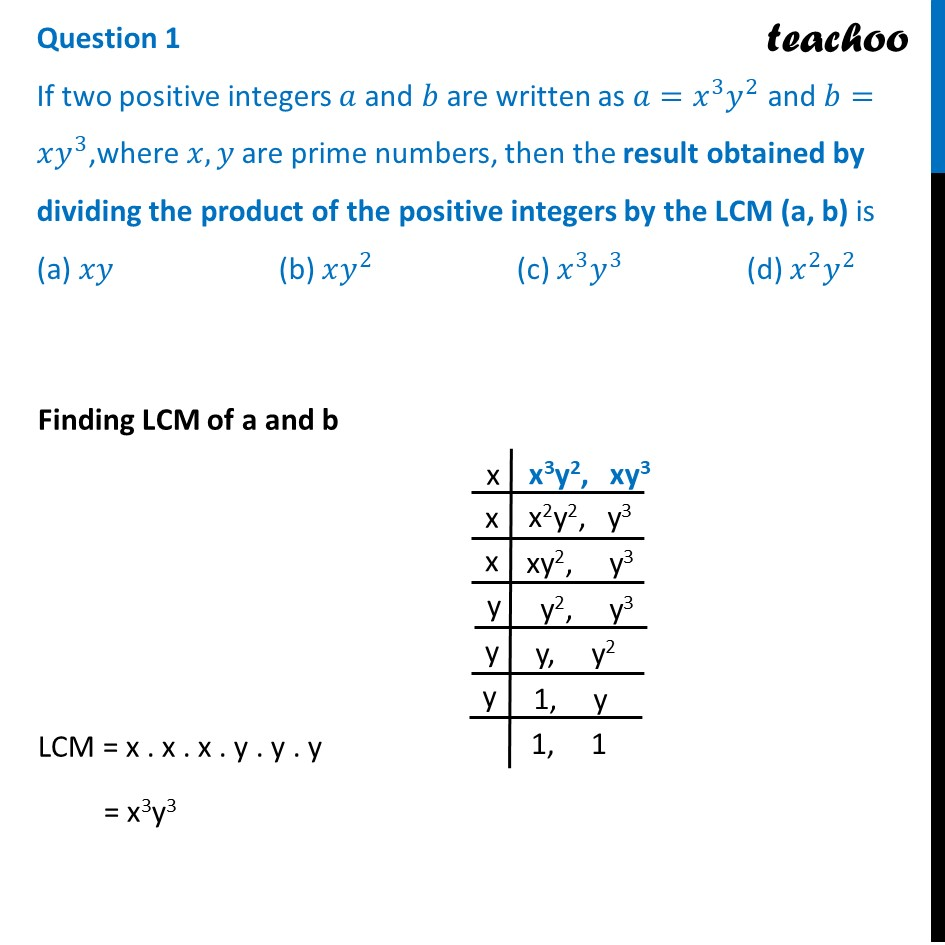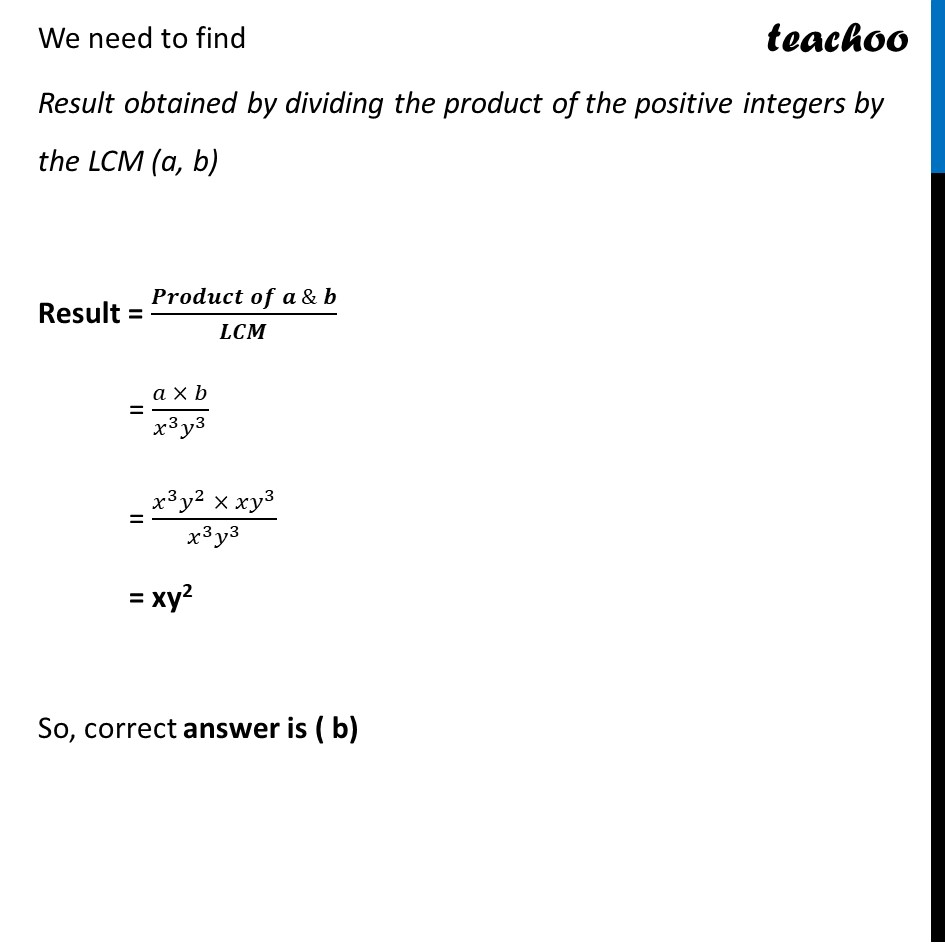CBSE Class 10 Sample Paper for 2024 Boards - Maths Standard

Class 10
Solutions of Sample Papers for Class 10 Boards

## (a) xy                       (b) xy^2                    (c) x^3 y^3                 (d) x^2 y^2

This Question is similar to Question 8 - MCQ from NCERT Exemplar - Chapter 1 Class 10 Real NumbersLearn in your speed, with individual attention - Teachoo Maths 1-on-1 Class

### Transcript

Question 1 If two positive integers 𝑎 and 𝑏 are written as 𝑎=𝑥^3 𝑦^2 and 𝑏=𝑥𝑦^3,where 𝑥,𝑦 are prime numbers, then the result obtained by dividing the product of the positive integers by the LCM (a, b) is (a) 𝑥𝑦 (b) 𝑥𝑦^2 (c) 𝑥^3 𝑦^3 (d) 𝑥^2 𝑦^2 Finding LCM of a and b LCM = x . x . x . y . y . y = x3y3 We need to find Result obtained by dividing the product of the positive integers by the LCM (a, b) Result = (𝑷𝒓𝒐𝒅𝒖𝒄𝒕 𝒐𝒇 𝒂 & 𝒃)/𝑳𝑪𝑴 = (𝑎 × 𝑏)/(𝑥^3 𝑦^3 ) = (𝑥^3 𝑦^2 × 𝑥𝑦^3)/(𝑥^3 𝑦^3 ) = xy2 So, correct answer is ( b)